# B.2 Interaction Picture

In the interaction representation both the state vectors and the operators are time-dependent. The state vector in the interaction representation is given by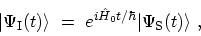(B.5)

which is merely a unitary transformation at the time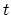. The equation of motion of this state vector is found by taking the time derivative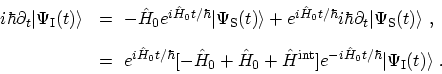(B.6)

Therefore, one obtains the following set of equations in the interaction picture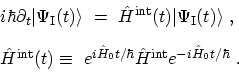(B.7)

An arbitrary matrix element in the SCHRÖDINGER picture can be written as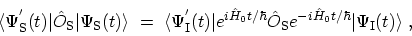(B.8)

which suggests the following definition of an operator in the interaction picture(B.9)

M. Pourfath: Numerical Study of Quantum Transport in Carbon Nanotube-Based Transistors